# NLU Visualization Examples

NLU can do quite a lot of things in just one line.
But imagine the things you could do in multiple lines, like visualizations!
In this section we will demonstrate a few common NLU idioms for the data science lifecycle, especially for the data exploration phase.

The most common two liner you will use in NLU is loading a classifier like emotion or sentiment and then plotting the occurence of each predicted label .

An few examples for this are the following :

emotion_df = nlu.load('sentiment').predict(df)
emotion_df['sentiment'].value_counts().plot.bar()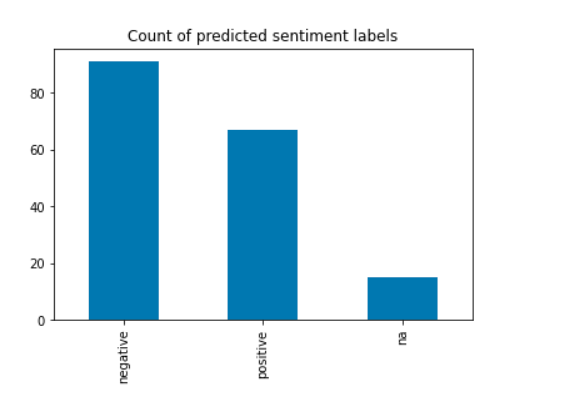emotion_df = nlu.load('emotion').predict(df)
emotion_df['emotion'].value_counts().plot.bar()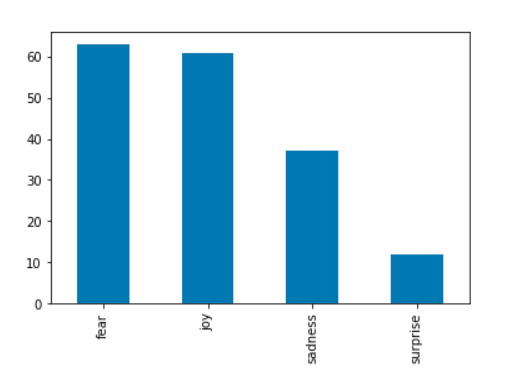Another simple idiom is to group by an arbitrary feature from the original dataset and then plot the counts four each group.

emotion_df = nlu.load('sentiment').predict(df)
sentiment_df.groupby('source')['sentiment'].value_counts().plot.bar(figsize=(20,8))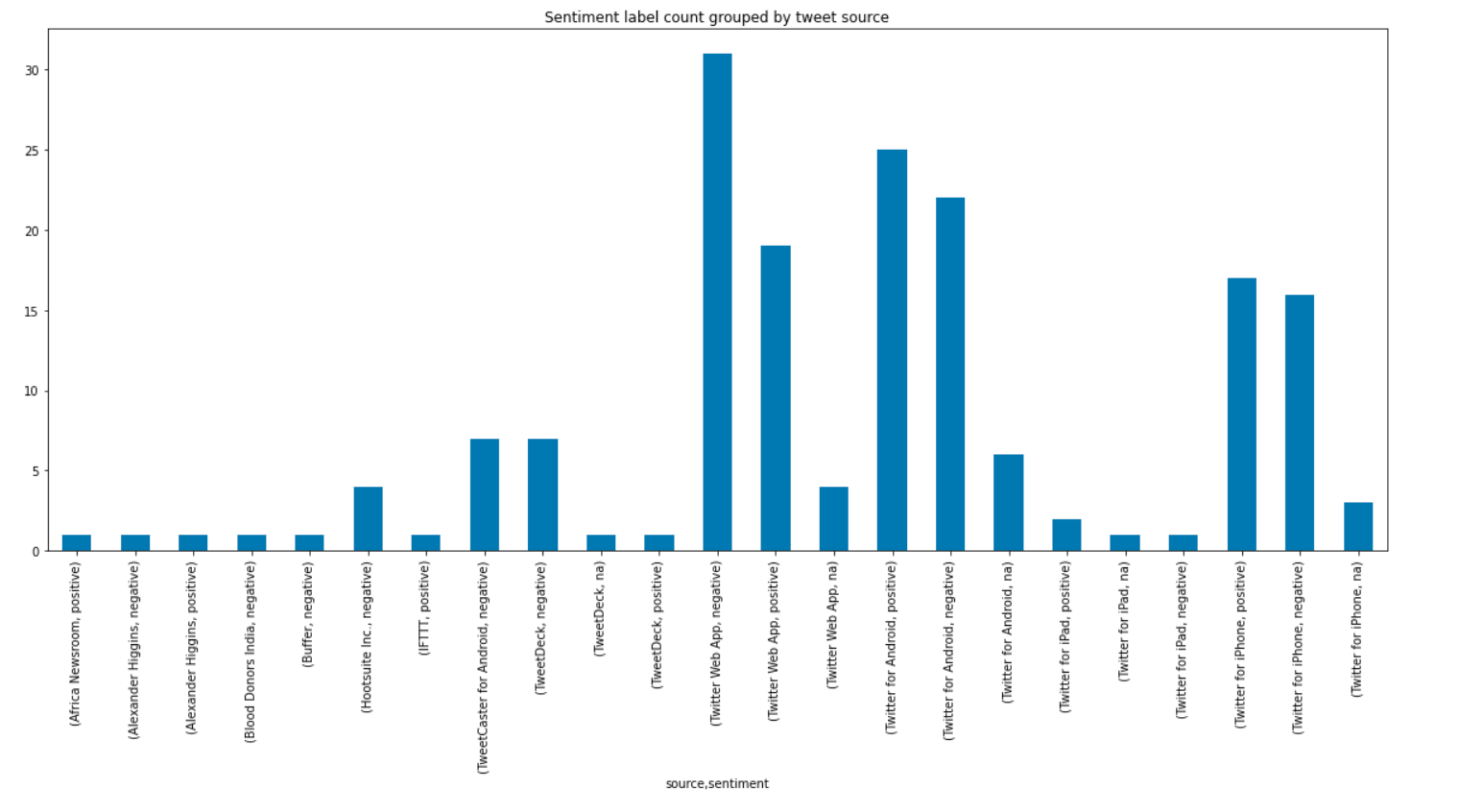nlu_emotion_df = nlu.load('emotion').predict(df)
nlu_emotion_df.groupby('airline')['emotion'].value_counts().plot.bar(figsize=(20,8))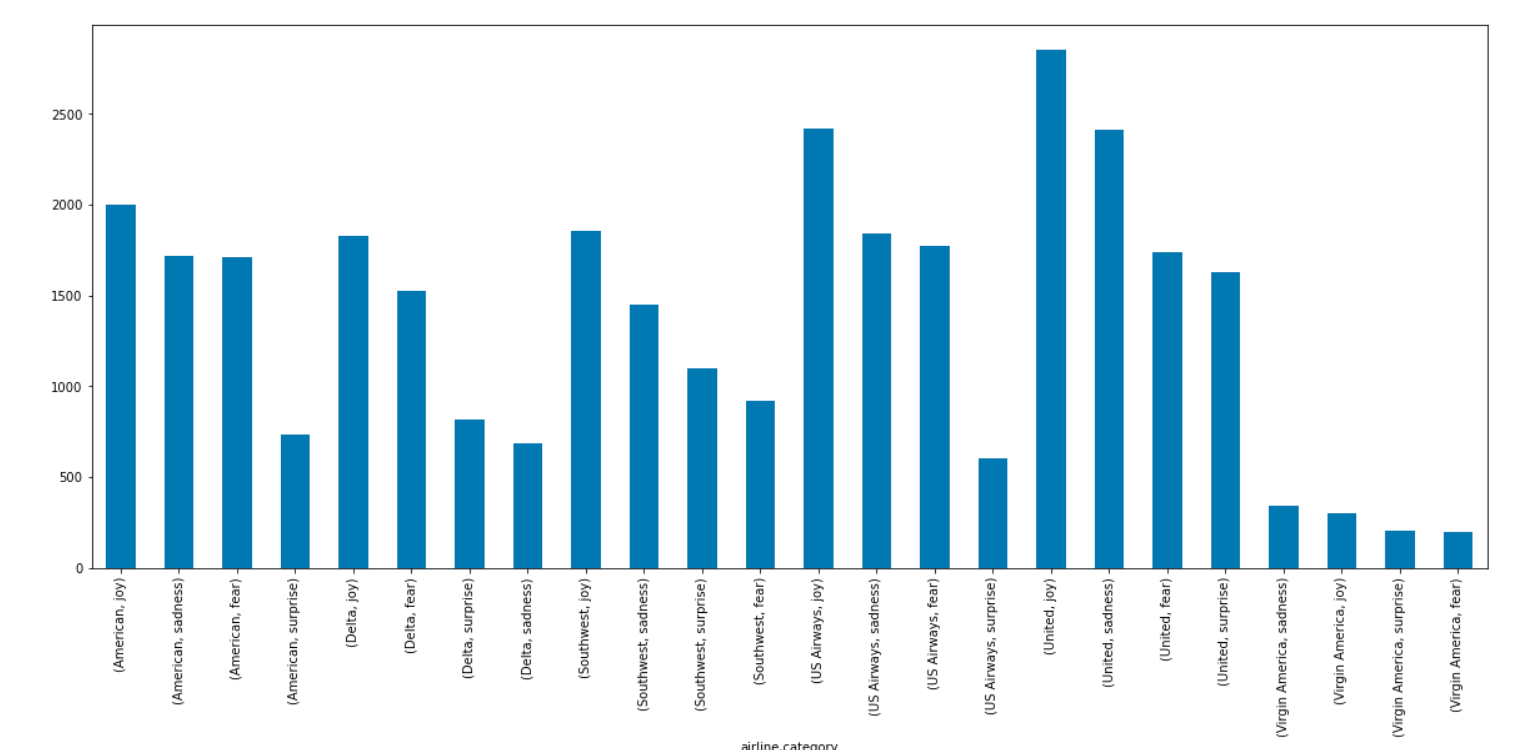You can visualize a Keyword distribution generated by YAKE like this

keyword_predictions.explode('keywords').keywords.value_counts()[0:100].plot.bar(title='Top 100 Keywords in Stack Overflow Questions', figsize=(20,8))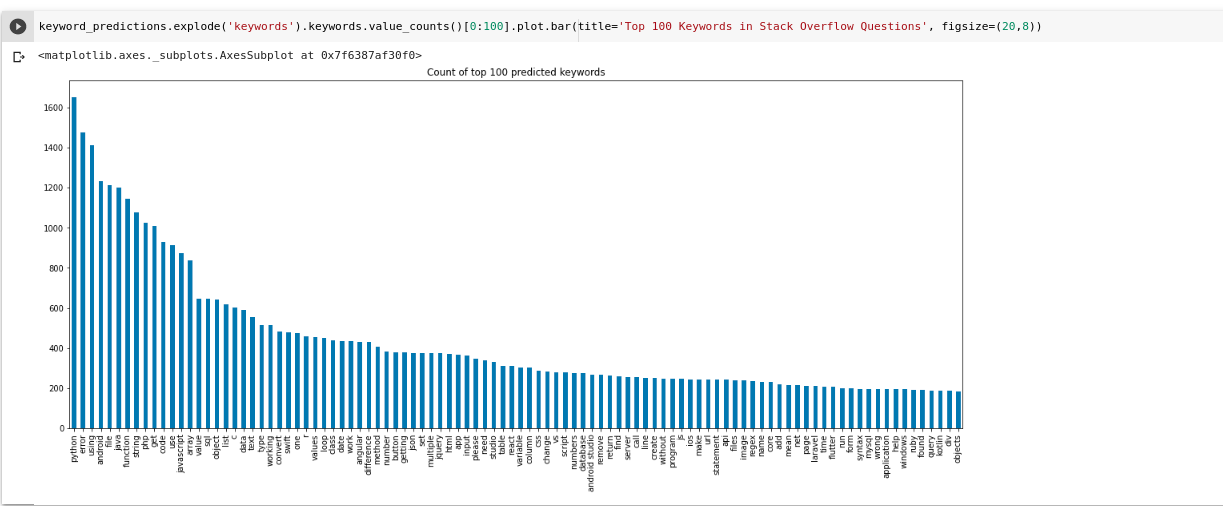Last updated